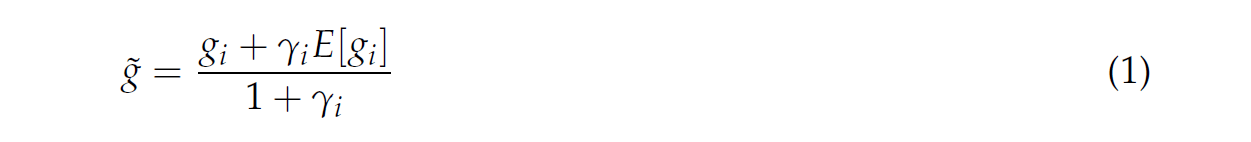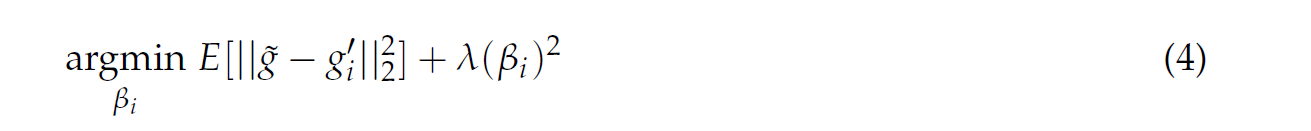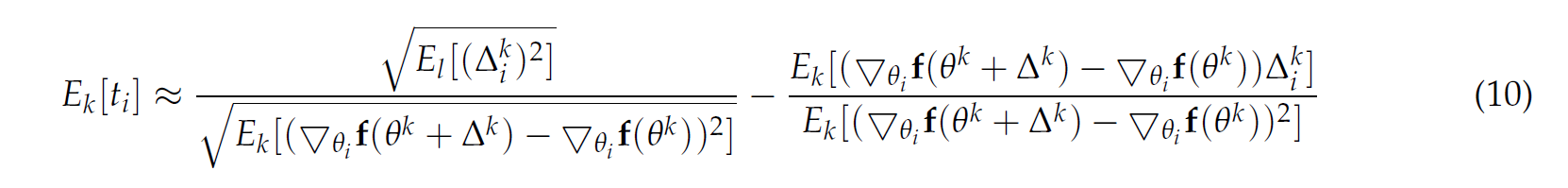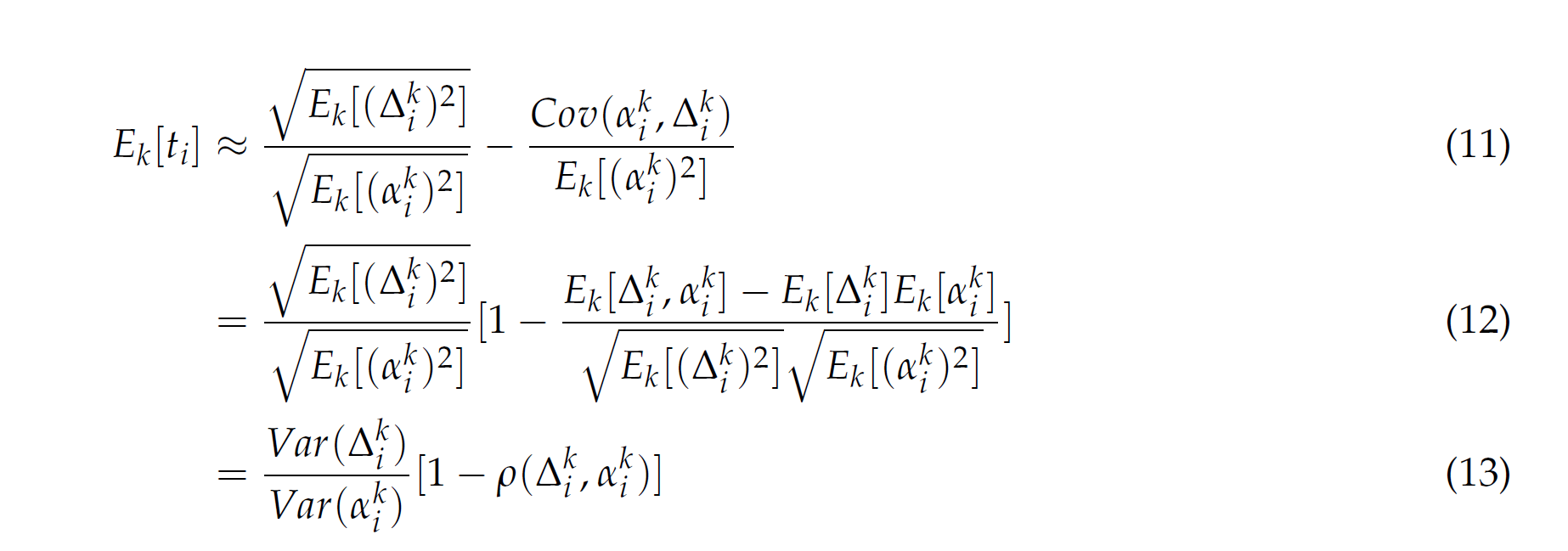This paper proposes an adaptive learning rate algorithm, which utilizes stochastic curvature information of the loss function for automatically tuning the learning rates.

Article source: https://arxiv.org/abs/1703.00788v1
Caglar Gulcehre, Jose Sotelo, Marcin Moczulski, Yoshua Bengio
(Submitted on 2 Mar 2017)

Stochastic gradient algorithms are the main focus of large-scale optimization problems, and have led to important successes in the recent advancement of the deep learning algorithms. The convergence of SGD depends on the careful choice of learning rate and the amount of the noise in stochastic estimates of the gradients. In this paper, we propose an adaptive learning rate algorithm, which utilizes stochastic curvature information of the loss function for automatically tuning the learning rates. The information about the element-wise curvature of the loss function is estimated from the local statistics of the stochastic first order gradients. We further propose a new variance reduction technique to speed up the convergence. In our experiments with deep neural networks, we obtained better performance compared to the popular stochastic gradient algorithms.I. Introduction
This paper presents a new stochastic gradient descent algorithm with adaptive learning rates. The learning rate update rule is estimated from stochastic curvature data of the loss function. In addition, this paper proposes a technique to reduce the variance of stochastic gradient estimator dependent only on the statistics related to the gradient itself.

II. Algorithm Update Rule
Before we dive into the paper. I will first introduce some gradient descent specific notation and terminology as used in the paper.For the proposed robust adaptive stochastic gradient descent method, a second-order directional Newton is developed to determine the step update △k.Where f is the objective function, △k is a unit vector of direction that the algorithm should descend in, ▽ is the gradient (vector differential) operator, and H is the Hessian matrix. Notice the difference between the step update using the proposed algorithm and Newton’s method, △k= -▽² f (θk)-¹ ▽ f (θk). Observe that the proposed algorithm involves a simple inversion of a diagonal matrix as to Newton’s method. The intuition behind this step update is immediate:

• we wish to travel in the direction opposite of the gradient, hence the negative of the gradient
• as for how much we descend in each direction, this is determined by the step tk, hence the diag(tk)
• this tis inversely proportional to the curvature (Hessian), hence the projection of dk onto H

The direction dk in a stochastic setting is chosen by using a block-normalized gradient vector which I will discuss in a later section.

III. Proposed Variance Reduction Technique
In the paper, a variance reduction technique is proposed for stochastic gradient descent that is dependent on basic statistics related to the gradient. Let gi denote the ith element of the gradient vector g with respect to the parameters q and expectation E[.] over minibatches. The proposed technique involves the following transformation:Note that E[ ˜ gi] = E[gi], and Var( ˜ g) = 1
(1+gi)2Var(gi). This is done by computing E[ ˜ g] and Var( ˜ g) =

E[( ˜ g – E[gi])2] where ˜ g is defined in (1). Therefore, the variance is reduced by a factor of (1 + gi)2.

The term E[gi] is not available in practice, therefore we will use a biased estimator ¯ g based on past values of gi. Let’s rewrite (1) as follows:Let βi = 1
1+gi
, (2) becomes:Let us observe that (3) is a convex combination of the unbiased, but high variance gi, and the biased, but low variance ¯ gi. We can adjust the weights of the two elements by changing βi (or gi). The proposed method to choose βi is by solving the following optimization problem:Equation (4) is convex in βi and has the following closed form solution:Therefore, we can see that as Var(gi) increases, gi increases, βi decreases. This means that the higher the variance that the gradient has, the lower the influence it will have when estimating ˜ g.

The blockwise gradient normalization is introduced to tackle the vanishing gradients problem. For any gradient based method, the parameters are learned by computing how a small perturbation in the parameters affect the output of the network. The vanishing gradient problem can be described as the gradients of the network’s output with respect to the parameters in the first few layers becoming extremely small. This causes the output to be insensitive to (large) changes in the input. As mentioned in the paper, this can be caused by repeated application of non-linearities. To interpret this, we can think of the activation functions in the neural network. The activation functions are often non-linearly map their inputs into a “small” range. This problem is worsened in neural networks with more layers.
Blockwise gradient normalization normalizes the gradients coming into each layer to have norm 1. The normalized gradient ˜g is computed as ˜g = g/(||E[g]||2), where E[g] is estimated using moving averages.

V. Choosing the Step-Size in Stochastic Case
The paper proposes a second order Taylor approximation of Ek[ti], which is the expectation of the step size over the minibatches. The equation for Ek[ti] is given as:Note that for small △ki , the denominator in the argument of the expectation above is approximately H-1k.
The proposed step size is approximated as:The paper proposes a further simplification of Ek[ti] as:I will try to give some intuition (to the best of my knowledge) of the monstrous equations (9) and (10).
Ek[ti] is the expectation of the step size which is ultimately used as the learning rate in the algorithm (see next section).And now, let  aki = ▽θi f(θk + △k) -▽θi f(θk). Let’s rewrite (9) and check out the following manipulation to help us understand:Where ρ(△ki , aki)  is the correlation between △ki and aki. Looking at (13), we can gain some intuition about the step size:

• if the step direction (defined above as a modified Newton’s step) has high variance, we wish to increase the step size
• if the curvature in the direction of the step has large variance, we wish to take smaller steps
• if the step direction and the curvature of the cost function are positively correlated, we wish to decrease the step size
• if the step direction and the curvature of the cost function are negatively correlated, we wish to increase the step size

The step sizes chosen above affect the convergence rate. For a fixed step direction △ki, draw a cost function at some point \theta^k (with different curvatures) and look in the step direction (should be a quadratic curve) to find the bottom of the curve. You will see that if the curvature of the cost function is large compared to the step direction, we want to take smaller steps to avoid overshoot.

VI. Algorithm
Now that we went over:

• the search direction △k at time k (section II)
• how to estimate the gradient g (section III)
• the step size tk at time k (section V)
• variance reduction for the gradient and tackle the vanishing gradient problem (section III, IV)

We look at the proposed algorithm:Which follows from the standard gradient descent algorithm, and since
we have already talked about everything except the red block, I will only give a brief description of this block as it is a more subtle point and not as important in understanding the algorithm.

First note the the tis just contributes to the weights of the moving average estimation of the gradient.
When a sample gradient is deemed to be an outlier (in this case if the difference between the sample gradient and the moving average estimation of the gradient is greater than two standard deviations, or same criteria but using the curvature), they reset τof the next step to be a constant 2.2. When τi= 2, we assign approximately the same amount of weight to the current and average of previous observations. I will not go into further detail since this is a very subjective modification to standard stochastic gradient descent methods. The calculation of τi at time k is found in the paper.
The results of this algorithm can be found in the original paper at link: https://arxiv.org/abs/1703.00788v1

VII. Conclusion
For this review, I went through some the main modifications of the proposed algorithm for stochastic gradient descent. I see the the main contributions of this paper as follows:
1) A simpler way of determining the step by using a directional secant approximation which involves a matrix inverse on a diagonal matrix (compared to a matrix inversion of the Hession matrix in Newton’s method)
2) A variance reduction technique to that is a convex combination of the sample gradient and an biased estimator of the expectation of the gradient
3) An adaptive way to select the step size using statistics about the curvature and gradient (compared to say,  line search used in many standard algorithms)
Finally, I hope by explaining how each parameter varies with the the information provided by the statistics of first order gradients and curvature will help you understand the algorithm better.

Author: Joshua Chou |Editor:  Junpei Zhong Localized by Synced Global Team: Xiang ChenMachine Intelligence | Technology & Industry | Information & Analysis

1.2.